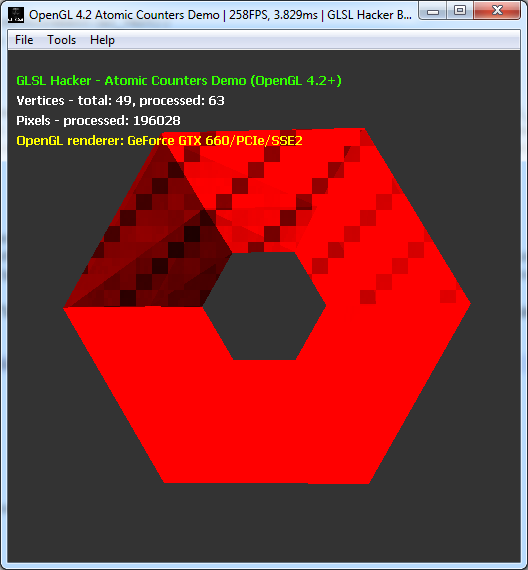# OpenGL 4.2 Atomic Counters: Rasterization Pattern, Helper for Rendering Optimization (Windows, Linux)

Article index:

## 2 – Number of Processed Vertices

A second use of the atomic counters is to get the number of vertices effectively processed by the vertex shader stage for rendering a mesh. For example, for a simple quad made up of 4 vertices, we will have 4 vertices effectively processed. But for more complex objects, the number of processed vertices is different from the number of vertices of the object.

Look at the following screenshot:The torus is made up of 49 vertices. But according to the atomic counter used to count the number of times the vertex shader has been executed, 63 vertices have been transformed. That means that 14 vertices have been transformed twice. In the case of GLSL Hacker, I would say it’s normal because there’s no particular mesh processing to take advantage of the GPU vertex cache (see Post Transform Cache for more details). Now if the vertex cache is correctly used, we should have a number of processed vertices smaller than the total number of vertices of the mesh.

Here is how to use the atomic counter in the vertex shader to count the number of processed vertices:

```#version 420
in vec4 gxl3d_Position;
layout(binding=1, offset=0) uniform atomic_uint ac_vert;
uniform mat4 gxl3d_ModelViewProjectionMatrix;
void main()
{
atomicCounterIncrement(ac_vert);
gl_Position = gxl3d_ModelViewProjectionMatrix * gxl3d_Position;
}
```

The atomic counter buffer (created with glGenBuffers, glBindBuffer and glBufferData + GL_ATOMIC_COUNTER_BUFFER) is bound the to second slot (binding=1) and starts at the offset 0 in this slot. In our case the size of the atomic counter buffer is 4 bytes (GLuint), it’s a single unsigned int. Several atomic counter buffers can be bound at the same time using different slots or binding points. You can specify the binding point of an atomic counter buffer with glBindBufferBase.

Once the rendering is done, you can read the content of the atomic counter with glMapBufferRange/glUnmapBuffer or with glGetBufferSubData.

More OpenGL code is available HERE or in the references at the end of the article.

Article index:

## 2 thoughts on “OpenGL 4.2 Atomic Counters: Rasterization Pattern, Helper for Rendering Optimization (Windows, Linux)”

1.zqueezy

nice work

2.John Smith